# Don't Quote Me: The Single and Double Quotes Problem

## 8 June 2001

As Gary Bergquist mentioned in the Limbering Up! column of the 2001 Q2 issue of his newsletter, now that some APL implementations allow both single and double quotes to mark a string, the natural question to ask by anyone who wants to parse such a line is which quote marks delimit the strings and which ones are inside a string.

While I am unable to find a simple solution, there is one which involves a very powerful (albeit less than practical) tool called Transitive Closure.

### Cut To The Chase

Give an arbitrary sequence of single and double quotes in (say) V, define the following variables

 I←(⍳⍴V)∘.=⍳⍴V Ye old identity matrix J←0,0 ¯1↓I Shift I one to the right (J is now a shift matrix) Q←<\((⍳⍴V)∘.<⍳⍴V)∧V∘.=V For each quote mark, what's the next matching one? R←Q⌹I-Q∨.∧J The 1st row marks the trailing quote in each pair
then

ZIRR.J

is the answer (or more accurately, the first row of that matrix is the answer).

It's easy to find simpler (and more efficient) ways of expressing this last line. However after we've used a matrix-divide on the previous line, the efficiency of the last line doesn't seem all that important.

Nonetheless, for the efficiency experts in the crowd, as you may already know, left-multiplying by J (that is, J.Q is equivalent to the expression (1 0Q)0 which shifts Q up one row (zero filling). Similarly, right-multiplying by J (Q.J) is equivalent to 0,0 ¯1Q, which shifts Q right one column (zero filling). Though the shifts are more efficient than matrix multiplication, it can be easier to understand the explanation below by leaving in J. Finally, as we're interested in only the first row of the resulting matrix, there's no need to perform those operations on the whole matrix R. Eventually, we're left with the last two lines of

R(V)QI-0,0 ¯1Q
ZR1,¯1R
.

### Doing It By Hand

As the matrix Q demonstrates, assuming that a given (single or double) quote mark is a string marker, it's easy to determine the matching trailing string marker. If we were solving this problem by hand, we would note that the first quote mark must be a string marker, and that the first row of Q gives us the identity of the matching trailing string marker. Having found one pair of string markers, we would move our finger over one spot to the next adjacent string marker (here's where we need that shift matrix), and start over again, chaining through the sequence of single and double quotes. Note that at the start of each iteration, we would use the row in Q corresponding to the index of the last trailing string marker plus one (the shift).

Finally, we need a mechanism to chain those assumptions together through the whole string, which is where Transitive Closure comes in.

### Transitive Closure

The term Transitive Closure comes from Graph Theory where it is used in the context of directed graphs. Assume we have an N by N boolean incidence matrix called M on the N nodes of a graph. These fancy terms mean simply that M[i;j] is 1 iff there is a directed edge from node i to node j. Transitive closure translates the concept "has a directed edge from i to j" to the concept "has a directed path of zero or more edges from i to j".

That is, if T is the transitive closure of M, then if M[i;j] and M[j;k], then T[i;k], and if M[i;j] and M[j;k] and M[k;l], then T[i;l], etc. In other words, transitive closure takes the concept of transitivity between edges (i is connected to j which is connected to k, therefore i is connected to k) to the limit (closure). For certain incidence matrices (in particular, they must be upper triangular), there is a closed form representation of transitive closure of the incidence matrix using power series.

Applying this to the problem at hand, Q gives us each matching quote mark, which we use to chain through the sequence of matches. By definition, the first quote mark delimits a string, and the 1st row of Q gives us the matching quote mark (say in the 5th position), the next (6th) quote mark begins the next pair, and the 6th row of Q gives us its matching quote mark, etc.

### An Infinite Set of Matrices

Concentrating on the sequence of trailing string markers, everything we need is in Q. Looking at each row of Q, it answers the question "If the quote mark corresponding to this row number starts a string, where is the matching trailing string marker?".

Ordinarily, when chaining from one element to another with transitive closure the expression Q.Q identifies the next marker, however in this case we must shift to the next string after finding a match so we need to shift the right hand matrix as in Q.J.Q. This expression answers the question "If the quote mark corresponding to this row number starts a string, where is the matching trailing string marker of the string next to this one?".

Putting this all together, the trailing string markers are identified as follows:

 Q The 1st row has the 1st trailing marker Q∨.∧J∨.∧Q The 1st row has the 2nd trailing marker Q∨.∧J∨.∧Q∨.∧J∨.∧Q The 1st row has the 3rd trailing marker Q∨.∧J∨.∧Q∨.∧J∨.∧Q∨.∧J∨.∧Q The 1st row has the 4th trailing marker
etc.

To get the whole result, we together these separate matrices as in

Q(Q.J.Q)(Q.J.Q.J.Q)(Q.J.Q.J.Q.J.Q)...

Note that throughout this discussion, we're taking advantage of the fact that . is associative.

The above expression can be simplified by factoring out Q, which we can do because . is also distributive. Note we can arbitrarily factor out Q on either the left or right; for reasons which will become clear below, I chose to factor it out on the right.

This leaves us with:

 I Q∨.∧J Q∨.∧J∨.∧Q∨.∧J Q∨.∧J∨.∧Q∨.∧J∨.∧Q∨.∧J
etc.

Joining these together gives

I(Q.J)(Q.J.Q.J)(Q.J.Q.J.Q.J)...

### Closed Forms

The sequence above is a power series, which is amenable to being rewritten in a closed form. Recall that a power series of the form

1 + X + (X×X) + (X×X×X) + (X×X×X×X) +...

can be expressed in closed form as

÷(1 - X)

for a suitable range of X. In the context of a power series, this closed form can be used more broadly on other objects including matrices with a suitable substitution of the functions and variables. In our case, we'll use the following substitutions:

1 is I,
+ is ,
X is Q.J,
× is ., and
÷ is .

Thus, the closed form of the power series can be expressed as

I-Q.J

We factored out Q earlier, so we need to multiply it back in again, which yields

R(I-Q.J).Q

or more simply (and here's why I factored out Q on the right)

RQI-Q.J

Had we factored out Q on the other side, we'd be left instead with the equivalent form

RQ.I-J.Q

As a side note, because we're interested in only the first row of R, this equivalent form can be written more simply as

RQ[1;].I-J.Q

In either case, the 1st row of R identifies all of the trailing string markers. As a side effect, if the last element in the 1st row of R is 1, then the string markers are all properly matched.

Finally, because another valid string marker (beginning the next string) follows every trailing string marker, a shift to the right of R (that is, R.J) gives the starting string markers (except for the 1st one which is where I comes in). Putting this all together, the final answer is the 1st row of

ZIRR.J

Bergquist actually requested something more, that is a function called NONQUOTE which extracts from a line of text just the characters which are outside strings.

Such a function can be written as follows:

```    ∇ R←NONQUOTE CV;N;V;Q
   ⍝ Returns all of CV except for quotes
   ⍝  or their contents.
   N←CV∊'''"' ⍝ Mask for quotes
   V←N/CV  ⍝ Select the quotes so we process less
   Q←<\((⍳⍴V)∘.<⍳⍴V)∧V∘.=V ⍝ Matching trailing string marker
   R←(⍴V)⍴Q⌹((⍳⍴V)∘.=⍳⍴V)-0, 0 ¯1↓Q ⍝ All trailing markers
   R←R∨1,¯1↓R ⍝ Both leading and trailing string markers
   R←(N⍱≠\N\R)/CV ⍝ Just the non-quoted material
∇```

### Fonts

If you have trouble displaying the APL characters on this page, likely it is due to either a browser setting (or an out-of-date browser) or a missing font. Both Mozilla Firefox 2.0 or later and Internet Explorer 7 or later display the APL characters perfectly, but IE6 has some trouble. If this page doesn't display well with either APL Unicode font using any version of Internet Explorer, please try it again with Mozilla Firefox. Links for the two APL Unicode fonts as well as for Mozilla Firefox appear at the top of this page.

### Author

This page was created by Bob Smith -- please any questions or comments about it to me. See my other APL projects.

This article can be found on the web at http://www.sudleyplace.com/APL/DontQuoteMe.ahtml.

### Acknowledgments

This code was developed and tested on APL+Win 3.6, courtesy of APL2000, Inc.

Gary Bergquist's quarterly Zark APL Tutor Newsletter comes as part of his Zark APL Tutor service; it can be ordered from him for \$29/year at

Zark Inc.
23 Ketchbrook Lane
Ellington, CT 06029
860-872-7806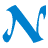NARS2000© 2006-2020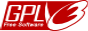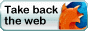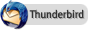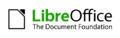Comments or suggestions? Send them to .